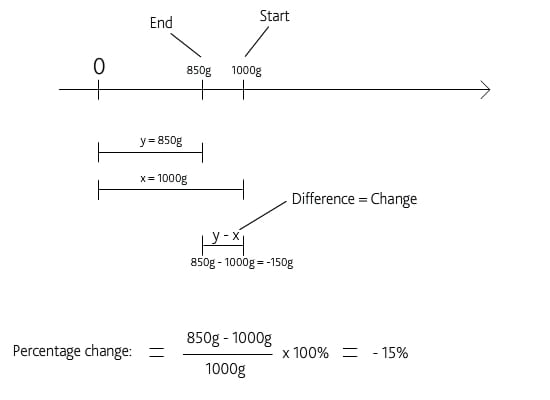# Percentage difference

This blogpost explains how to understand and calculate percentage change as this calculation is part of the SCAE certification system on the roast log template you can download: here

So let us get right go business:
If a process changes from x to y the percentage of change is referring to how big the change is seen in relationship to where the process ‘came from’ namely x.

So the general formula for a percentage change is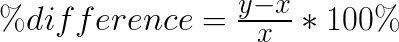$latex.php$

If you are not used to do these kinds of calculations, I would like to explain you this formula in more detail as follows:

In the figure below you see a process that goes from value x to y (could be an increasing temperature during roasting) and you can see how you can calculate the difference between the starting point of the process to the endpoint of the process by subtracting x from y.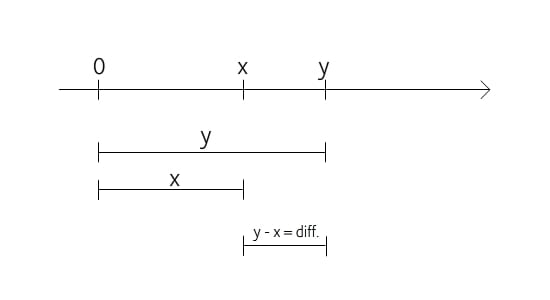Let us take an example. You started you coffee roastery 12 months ago and you currently you have 15 customers. After 9 months you had 10 customers. How many more customers do you have now compared to when you business was 9 months old? In other words: what is the difference between the number of customers you have now compared to when the company was 9 months old: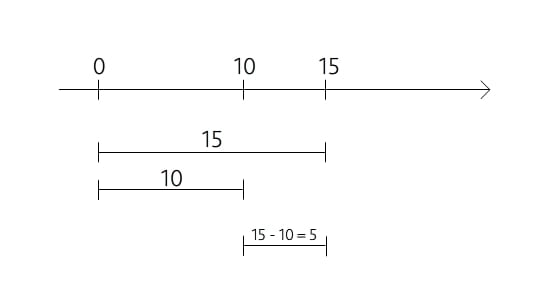So in absolute numbers of customers this is 5 more than after 9 months.

But how to calculate this value as a percentage?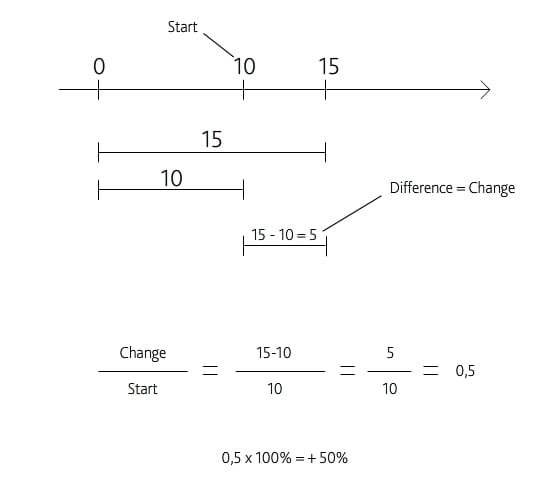As you can see from the above calculation, you relate the change (5 customers difference) to the starting point of comparison (10 customers after 9 months) by deviding the change with the starting point of comparison. And as you can also see from the above figure the value is +50% which is a positive number since the process increased in the period.
So the following figure shows you the general formula for the above calculation: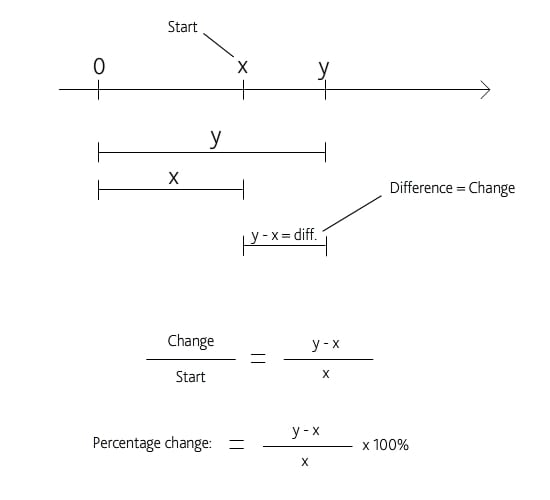But what happens if you monitor a process that is decreasing? Namely where y is smaller than x because the process is decreasing. This is illustrated graphically here: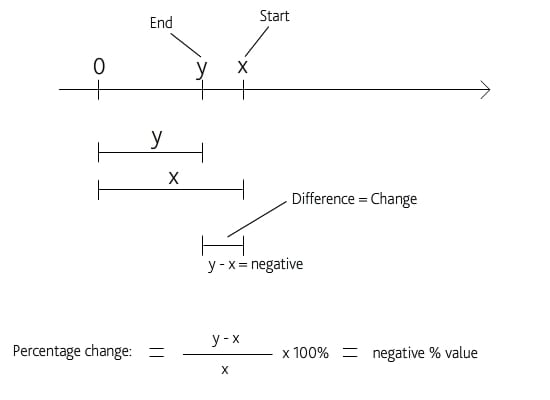The value of y – x becomes negative because x is bigger then y so a decreasing process would give you a negative change and if you have a negative change that you divide with the starting point of comparison you also get a negative percentage.

In the following example we look at roast loss which is a process where you compare the result of the roast (y) with the initial amount of coffee you added (x) and find a negative value for the percentage of change. Let us assume that we put in 1kg (1000g) into a roaster and perform a light roast and measure the weight of the roasted coffee (when calculating roast lost please remember to NEVER remove any beans with the sample spoon during the roast!) and find out that 850g of roasted coffee came out of 1000g of green. The calculation looks like this:So the percentage of change is -15% which roast masters would refer to as 15% roast loss since the word ‘loss’ implies a negative change.

#### Morten Münchow

Founder of CoffeeMind. Coffee roaster, educator/trainer and researcher. Read more here

This Post Has One Comment
1.weilhalt says:

Or use the rule of three: 1000g = 100%, 850g = x% -> x=85%